The SURVEYSELECT Procedure

### Proportional Allocation

When you specify the ALLOC=PROP option, PROC SURVEYSELECT allocates the total sample size among the strata in proportion to the stratum sizes, where the stratum size is the number of sampling units in the stratum. The allocation proportion of the total sample size for stratumequals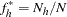where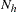is the number of sampling units in stratumand
is the total number of sampling units for all strata. Based on this allocation proportion, the target sample size for stratumis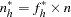whereis the total sample size that you specify in the SAMPSIZE= option.

The target sample size values,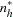, might not be integers, but the stratum sample sizes must be integers. PROC SURVEYSELECT uses a rounding algorithm to convert theto integer values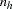and maintain the requested total sample size. The rounding algorithm includes the restriction that all values ofmust be at least 1, so that at least one unit will be selected from each stratum. For without-replacement selection methods, PROC SURVEYSELECT also requires that each stratum sample size must not exceed the total number of sampling units in the stratum,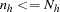. If a target stratum sample size exceeds the number of units in the stratum, PROC SURVEYSELECT allocates the maximum number of units,, to the stratum, and then allocates the remaining total sample size proportionally among the remaining strata.

PROC SURVEYSELECT provides the target allocation proportions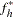in the output data set variable AllocProportion. The variable ActualProportion contains the actual proportions for the allocated sample sizes. For stratum, the actual proportion is computed as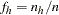whereis the allocated sample size for stratum, andis the total sample size. The actual proportions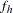can differ from the target allocation proportionsdue to rounding and the restrictions that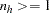and.Previous Page | Next Page | Top of Page